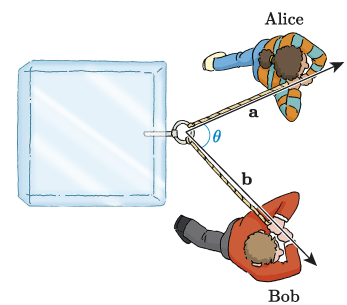Science, Maths & Technology

### Become an OU studentIntroducing vectors for engineering applications

Start this free course now. Just create an account and sign in. Enrol and complete the course for a free statement of participation or digital badge if available.

# 4.4 Finding the angle between two vectors

The scalar product of two vectors has an important application in calculating the angle between two vectors. If we start with the definition of the scalar product in terms of the magnitudes and directions of the vectors, and rearrange it, then we get the following result.

## Angle between two vectors

The angle between any two non-zero vectors and is given by

We can use this result to find the angle between two vectors in component form.

## Example 8 Calculating the angle between two vectors in component form

Alice and Bob have attached ropes to a face of the block of ice and are pulling it in different directions, see Figure 34. Vector describes the force applied by Alice, and in component form is given by . Vector describes the force applied by Bob, and in component form is given by .

What is the angle between these vectors, to one decimal place?Figure 34 Alice and Bob pulling a block of ice

### Solution

First let’s use the components of and to find , and . We have

Using these we can calculate :

So

Therefore the angle between the vectors is 64.4° (to 1 d.p.).

## Activity 22

Find, to the nearest degree, the angle between the vectors